# How to Convert Decimal Number to Binary/Octal/Hex Number or Vice Versa in Excel?

You might occasionally have needed to convert a decimal number in Excel into another format, such as binary, octal, or hexadecimal. In general, decimal numbers have a base of 10 from 0 to 9, binary numbers have a base of 2 from 0 to 1, octal numbers have a base of 8 from 0 to 7, and hexadecimal numbers have a base of 16 from 0 to 9 and A to F. You may learn how to convert decimal numbers in Excel to binary, octal, and hexadecimal by reading this article.

## Convert Decimal Number to Binary Number in Excel

Here, we will create a list of decimal numbers, then use the formula to convert the numbers to other formats, and finally use the autofill handle to complete the process. Let us look at a simple procedure for converting a decimal number to a binary number in Excel using formulas. We can do it very simply by using the formulas.

### Step 1

Let us consider an Excel sheet where the data in the sheet is similar to the data shown in the below image.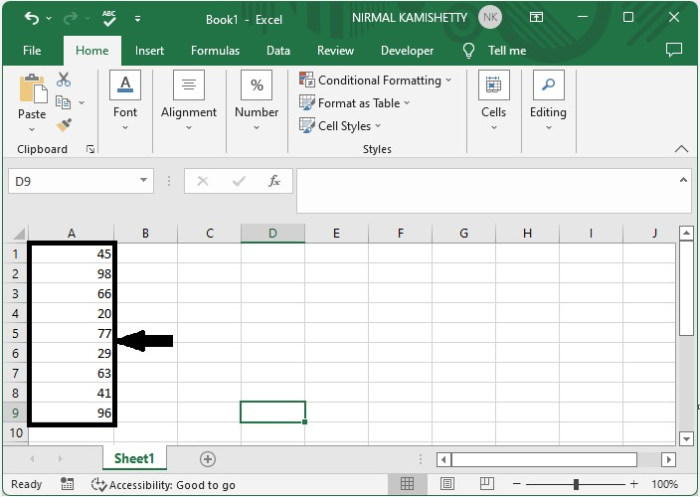To get our first result, click on an empty cell, in this case cell B1, enter the formula =DEC2BIN(A1), and press Enter.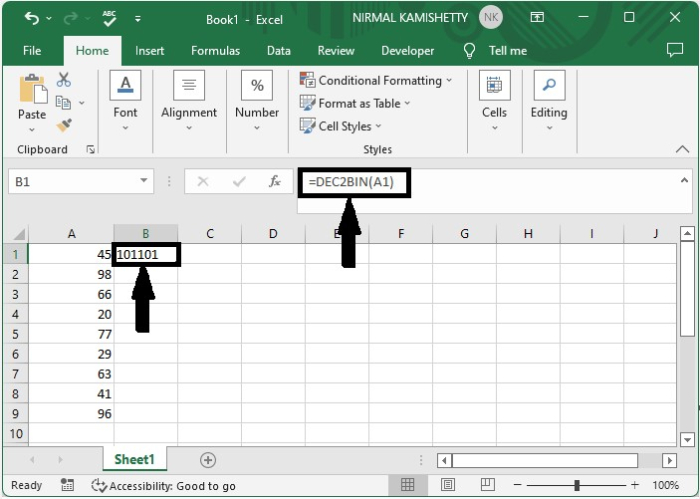### Step 2

We get all the other results by dragging down from the first result using the auto-fill handle, and our final result will be similar to the below image.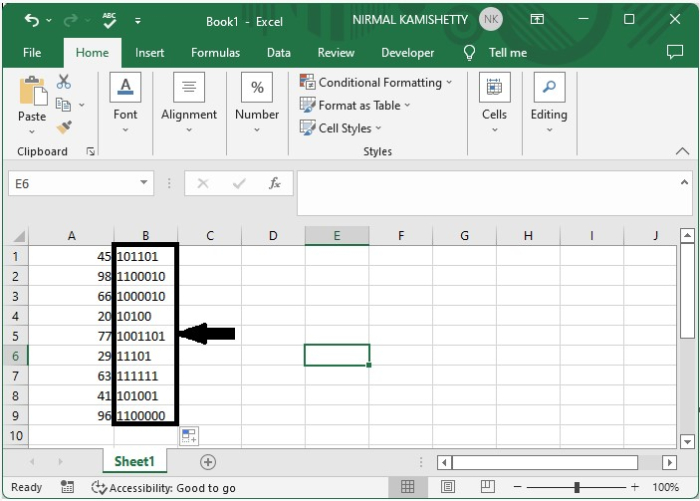When converting binary to decimal, we can use the formula =BIN2DEC(A1).

## Convert Decimal Number to Octal Number in Excel

Here, we will create a list of decimal numbers, then use the formula to convert the numbers to other formats, and finally use the autofill handle to complete the process. Let us look at a simple procedure for converting a decimal number to an octal number in Excel using formulas.

Consider the same data as in the previous example and click on an empty cell to enter the formula =DEC2OCT(A1) and press enter to get our first result.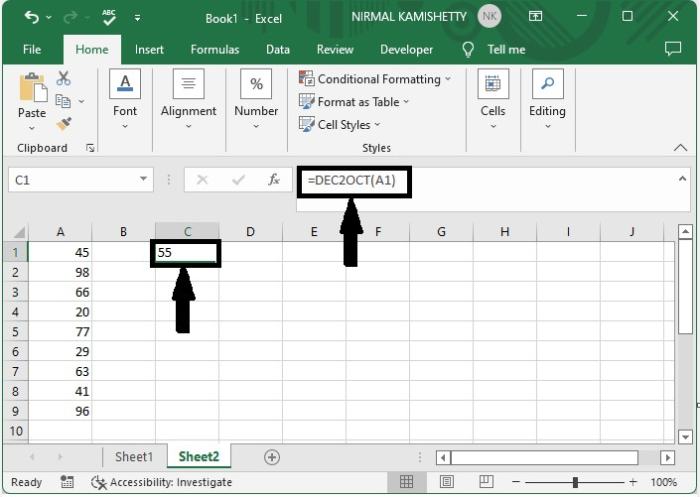We can get all the other results by dragging down from the first result. We can use the formula =OCT2DEC(A1) to convert octal to decimal.

## Convert Decimal Number to Hexadecimal in Excel

Here, we will create a list of decimal numbers, then use the formula to convert the numbers to other formats, and finally use the autofill handle to complete the process. Let us look at a simple procedure for converting a decimal number to hexadecimal in Excel using formulas.

Let us consider the same data that we used in the above example and click on an empty cell, enter the formula as =DEC2HEX(A1), and click enter to get our first result.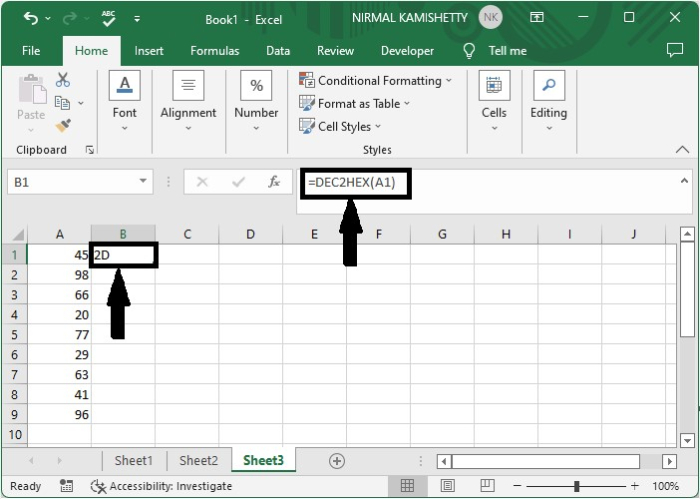We can get all the other results by dragging down from the first result. We can use the formula =HEX2DEC(A1) to convert hexadecimal to decimal.

## Conclusion

In this tutorial, we used a simple example to demonstrate how we can convert a decimal number to hex, decimal, or octal and vice versa in Excel to highlight a particular set of data.